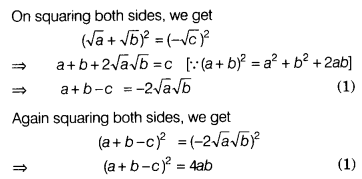# If √a + √b + √c = 0, then prove that (a + b-c)2 =4ab

If √a + √b + √c = 0, then prove that (a + b-c)2 =4ab.

We have √a + √b +√c =0
⇒ √a + √b = -√c